# 1. 主要观点

• 线性模型是线性回归和线性分类的基础
• 线性回归和线性分类模型的差异主要在于损失函数形式上，我们可以将其看做是线性模型在多维空间中“不同方向”和“不同位置”的两种表现形式
• 损失函数是一种优化技术的具体载体，影响损失函数不同形式的因素主要有：
• 和谁比：和什么目标比较损失
• 怎么比：损失比较的具体度量方式和量纲是什么
• 比之后如何修正参数：如果将损失以一种适当的形式反馈给原线性模型上，以修正线性模式参数

# 2. 线性模型，线性回归和线性分类的基础

## 0x1：线性模型中的“线性”指的是什么

Y = a1x1 + a2x2 + ..... + anxn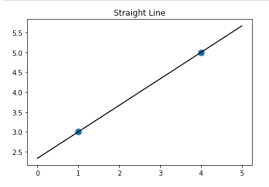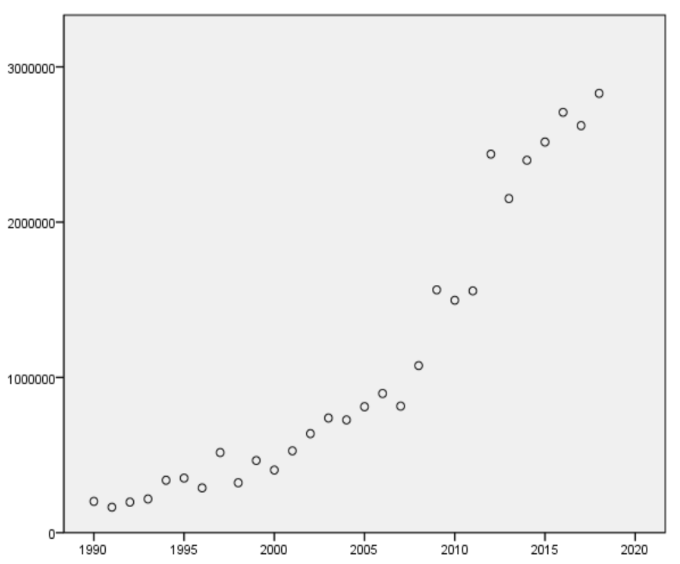# 3. 线性回归（linear regression）模型

## 0x1：线性回归的发展起源

“回归”是由英国著名生物学家兼统计学家高尔顿(Francis Galton,1822～1911，生物学家达尔文的表弟)在研究人类遗传问题时提出来的。• 当父亲高于平均身高时，他们的儿子身高比他更高的概率要小于比他更矮的概率；
• 父亲矮于平均身高时，他们的儿子身高比他更矮的概率要小于比他更高的概率

## 0x2：线性回归定义

• 一个地区的房价：由面积、地段、层数、周边配套等因素线性组成
• 孩子的身高：由父亲和母亲的身高两个因素线性组成

y = w0 + w1a1 + .... + wkak

## 0x3：从一元线性回归说起

### 1. 一个广告公司销售额回归预测问题### 2. 这条线是怎么画出来的？

Y = ax + b（a、b分别代表2个参数，是我们需要通过观察数据计算得到的）

### 3. 线性回归的损失函数必须选择最小二乘损失！高斯如是说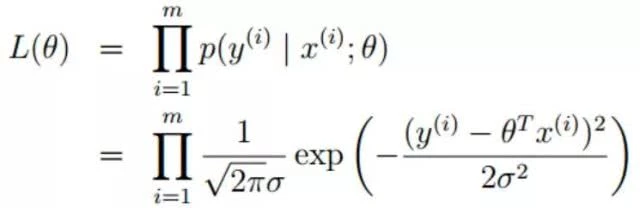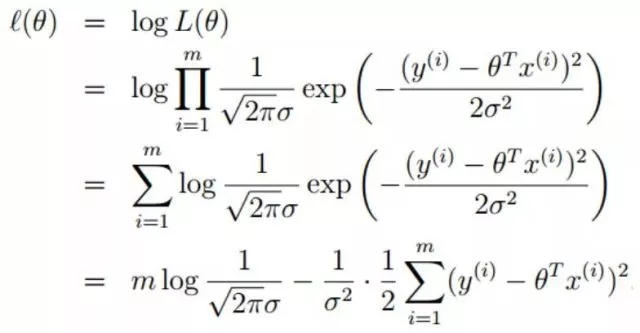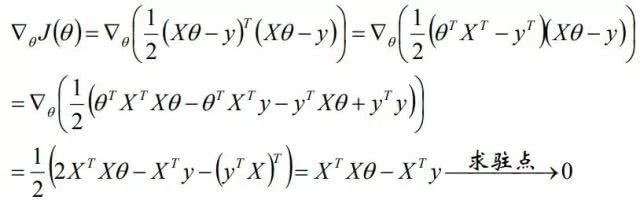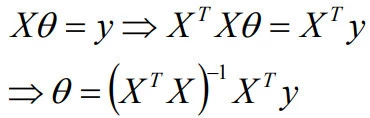Y=1.98X+2.25

### 5. 为什么对历史数据拟合的看起来不是那么准？

• 数据集本身存在异常噪音点（noise data）：样本本身可能存在一些“离群异常点”，这些点本身不存在任何线性相关关系。
• 数据集中包含非线性数据（non linear problem）：这可能是个严重的问题，意味着我们的建模就错误了，目标问题根本就不是一个线性关系问题，强行用线性模型拟合肯定是不行的。
• 异质性（欠特征化）：导致因变量的自变量不在我们选取的特征中，换句话说，即我们选择的特征不能很好的体现出线性型的特征。对于销售额这个例子来说，其实广告费只是影响销售额的其中一个比较重要的因素，可能还有经济水平、产品质量、客户服务水平等众多难以说清的因素在影响最终的销售额，那么实际的销售额就是众多因素相互作用最终的结果，由于销售额是波动的，回归线只表示广告费一个变量的变化对于总销售额的影响，所以必然会造成偏差，回归线只能解释了一部分影响。

### 6. 两个新的评价指标

SST（总偏差）= SSR（回归线可以解释的偏差）+ SSE（回归线不能解释的偏差）

• 总偏差平方和（SST，Sum of Squaresfor Total）：是每个因变量的实际值（给定点的所有Y）与因变量平均值（给定点的所有Y的平均）的差的平方和，即，反映了因变量取值的总体波动情况。实际上也就是方差。

• 回归平方和（SSR，Sum of Squares forRegression）因变量的回归值（直线上的Y值）与其均值（给定点的Y值平均）的差的平方和，即，它是由于自变量x的变化引起的y的变化，反映了y的总偏差中由于x与y之间的线性关系引起的y的变化部分，是可以由回归直线来解释的
• 残差平方和（又称误差平方和，SSE，Sum of Squaresfor Error）：因变量的各实际观测值(给定点的Y值)与回归值（回归直线上的Y值）的差的平方和，它是除了x对y的线性影响之外的其他因素对y变化的作用，是不能由回归直线来解释的

R= SSR/SST 或 R= 1-SSE/SSTR2的取值在0，1之间，越接近1说明拟合程度越好。

• 假如所有的点都在回归线上，说明SSE为0，则R2=1，意味着Y的变化100%由X的变化引起，没有其他因素会影响Y，回归线能够完全解释Y的变化
• 如果R2很低，说明Y的变化不完全由X的波动决定，X和Y之间可能不存在线性关系

• R=1：说明X和Y完全正相关，即可以用一条直线，把所有样本点（x,y）都串起来，且斜率为正
• R=-1：说明完全负相关，及可以用一条斜率为负的直线把所有点串起来
• R=0：则说明X和Y没有线性关系，注意，是没有线性关系，说不定有其他关系(例如非线性关系)

http://scikit-learn.org/stable/auto_examples/linear_model/plot_ols.html#sphx-glr-auto-examples-linear-model-plot-ols-py
http://www.jianshu.com/p/fcd220697182
https://baike.baidu.com/item/%E4%B8%80%E5%85%83%E7%BA%BF%E6%80%A7%E5%9B%9E%E5%BD%92%E9%A2%84%E6%B5%8B%E6%B3%95

## 0x4：扩展到多元线性回归

### 1. 多元线性模型数学公式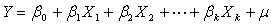•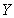被解释变量
•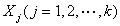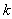解释变量
•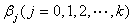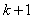未知参数
•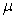随机误差项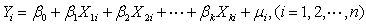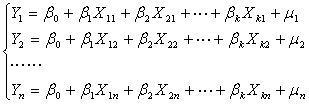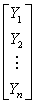=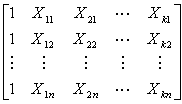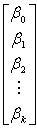+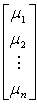•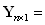被解释变量的观测值向量
•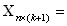解释变量的观测值矩阵
•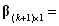总体回归参数向量
•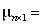随机误差项向量

### 2. 根据训练样本(观测数据)来推测出多元线性模式的参数 - 最大似然估计思想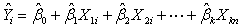•参数估计值
•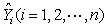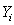样本回归值样本拟合值样本估计值

•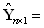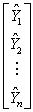拟合值列向量
•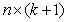样本观测矩阵
•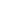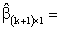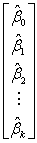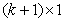参数估计值列向量

### 3. 多元线性模型下怎么评估拟合程度的好坏？

#### 1）残差平方估计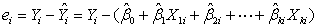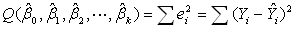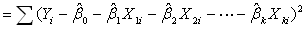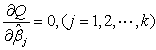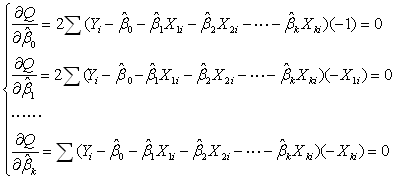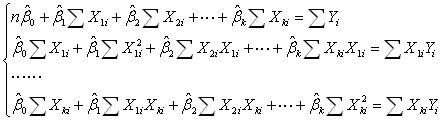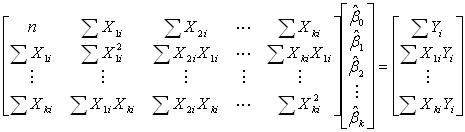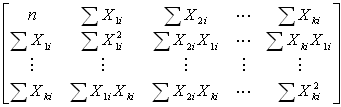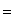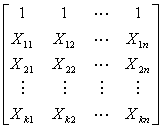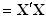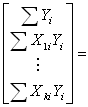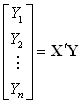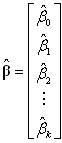为参数估计值向量，根据上式可得到正规方程组：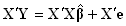#### 2）可决系数R2$R^2=\frac{\sum(\hat{y}-\bar{y})^2}{\sum(y-\bar{y})^2}$$=1-\frac{\sum(y-\hat{y})^2}{\sum(y-\bar{y})^2}$

#### 3）估计标准误差$S_y=\sqrt{\frac{\sum(y-\hat{y})^2}{n-k-1}}$

http://scikit-learn.org/stable/modules/generated/sklearn.linear_model.LinearRegression.html
https://medium.com/towards-data-science/simple-and-multiple-linear-regression-in-python-c928425168f9https://baike.baidu.com/item/%E5%A4%9A%E5%85%83%E7%BA%BF%E6%80%A7%E5%9B%9E%E5%BD%92%E6%A8%A1%E5%9E%8B
http://www.cnblogs.com/dfcao/p/ng_ml_2.html
http://www.cnblogs.com/zgw21cn/archive/2008/12/24/1361287.html
https://onlinecourses.science.psu.edu/stat501/node/311
http://wiki.mbalib.com/wiki/%E5%A4%9A%E5%85%83%E7%BA%BF%E6%80%A7%E5%9B%9E%E5%BD%92%E9%A2%84%E6%B5%8B

# 4. 线性分类（linear classification）模型

## 0x1：从多响应线性回归（multiresponse linear regression）说起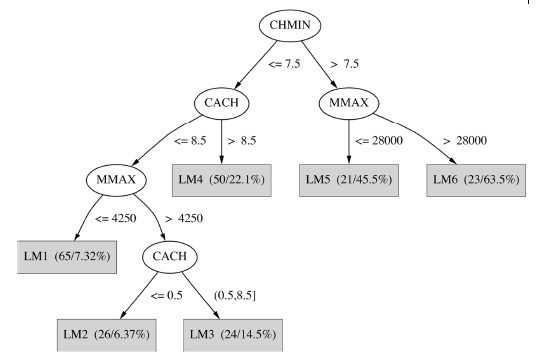• 对于属于这个类的实例，隶属函数值为1
• 对于其他类的实例，函数值为0

• 第一、隶属函数产生的不是概率值，从属关系值可能落在0~1之外
• 第二、最小二乘回归假设误差之间彼此独立，所有误差综合起来得到一个标准正态分布，当多响应线性回归用于分类问题时，明显违背了这个假设，因为这时观察值仅呈现0和1，是一种阶跃分布

## 0x2: 逻辑斯蒂回归（Logistic regression）分类模型

### 1. 逻辑斯蒂分布（logistic distribution）模型对线性回归（linear regression）的改机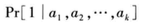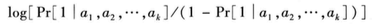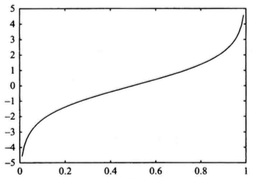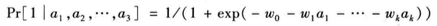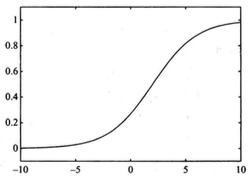### 2. Logistic regression模型概率公式• 形状参数r的值越小，曲线在中心附近增长得越快，也即越接近阶跃函数
• 阶跃点由位置参数u决定

### 4. 逻辑斯蒂回归模型参数估计

logistic regression模型建立后，接下来如何去求解模型中的参数。在统计学中，常常使用极大似然估计法来求解，即找到一组参数，使得在这组参数下，我们的训练样本数据被正确分类的似然度（概率）最大。### 5. 多项式逻辑斯蒂回归（多项代表label有多种可能）http://blog.csdn.net/xbinworld/article/details/43919445
http://blog.sciencenet.cn/blog-427701-688352.html
http://blog.csdn.net/ppn029012/article/details/8775597
http://blog.csdn.net/richard2357/article/details/17241039
http://blog.sciencenet.cn/blog-508318-633085.html
http://blog.csdn.net/daunxx/article/details/51816588
https://chenrudan.github.io/blog/2016/01/09/logisticregression.html
http://www.52cs.org/?p=286
https://yoyoyohamapi.gitbooks.io/mit-ml/content/%E7%BA%BF%E6%80%A7%E5%9B%9E%E5%BD%92/articles/%E5%9B%9E%E5%BD%92%E9%97%AE%E9%A2%98.html

## 0x3：感知机，一种简单的逻辑回归模型

logistic回归试图通过将训练数据的概率最大化的方法，产生正确的概率估计。现在假设只有两个类，如果使用一个超平面能够将数据完美地分成两组，那么就称该数据为线性可分（linearly separable）数据

## 0x4: 最大熵模型，逻辑斯蒂回归的一般泛化形式

### 1. 最大熵原理，其中| X |代表X的取值个数，当且仅当X的分布是均匀分布时右边的等号成立，即当X服从均匀分布时，熵最大。

• π(x)u输入时

### 3. 最大熵模型和逻辑斯蒂模型联系与区别总结

• 逻辑回归跟最大熵模型没有本质区别。逻辑回归是最大熵对应类别为二类时的特殊情况
• 概率函数指数簇分布的最大熵等价于其指数形式的最大似然函数
• 二项式分布的最大熵解等价于二项式指数形式(sigmoid)的最大似然函数
• 多项式分布的最大熵等价于多项式分布指数形式(softmax)的最大似然函数

http://blog.csdn.net/cyh_24/article/details/50359055

# 5. 从函数优化这个统一视角看线性回归和线性分类模型的区别与联系

## 0x1：从输入输出角度看"回归"和"分类"的区别

• 回归模型: 给定一个新的输入特征，推断它所对应的输出y(连续值实值)是多少, 也就是使用y=g(x)来推断任一输入x所对应的输出值。
• 分类模型: 给定一个新的输入特征, 推断它所对应的类别(大多数情况是二分类如: +1, -1，也可以基于二分类扩展出多分类), 也就是使用y=sign(g(x))来推断任一输入x所对应的类别。注意，模型的输出结果类别是有限的

## 0x2：从损失评估标准角度看"回归"和"分类"的区别

• 线性回归模型的损失评估是：(输出值) compare (输入值)
• 线性分类模型的损失评估是：(输出值) compare (F(输入值))，这里F(输入值)指依照某个标准将输入值映射为某些特定的类值

## 0x3：从决策超平面空间分布角度看"回归"和"分类"的区别

• 线性回归方程组的权重向量超平面是一个”靠近“数据集的超平面
• 线性分类方程组的权重向量超平面是一个”原理“数据集中不同类别的超平面决策面

## 0x4：回归和分类各自的应用场景

• 回归问题的应用场景 - 回归是对真实值的一种"逼近预测"
• 回归问题通常是用来预测一个值，如预测房价、未来的天气情况等等
• 例如一个产品的实际价格为500元，通过回归分析预测值为499元，我们认为这是一个比较好的回归分析
• 预测明天的气温是多少度，这是一个回归任务
• 分类问题的应用场景 - 分类并没有逼近的概念，最终正确结果只有一个，错误的就是错误的，不会有相近的概念
• 分类问题是用于将事物打上一个标签，通常结果为离散值
• 例如判断一幅图片上的动物是一只猫还是一只狗)
• 预测明天是阴、晴还是雨，就是一个分类任务，但是很容易理解

http://blog.csdn.net/caroline_wendy/article/details/13294893
https://my.oschina.net/zzw922cn/blog/544221?p=1
http://www.jianshu.com/p/d6f206c869ed
http://zh.numberempire.com/derivativecalculator.php
https://www.zhihu.com/question/21329754
https://hellozhaozheng.github.io/z_post/%E6%9C%BA%E5%99%A8%E5%AD%A6%E4%B9%A0-%E9%80%BB%E8%BE%91%E5%9B%9E%E5%BD%92/

# 6. 从鸢尾花例子看回归和分类问题的共性和区别

## 0x1: 前2维特征+4维PCA降维到3维的可视化数据集展示

# -*- coding: utf-8 -*-

import matplotlib.pyplot as plt
from mpl_toolkits.mplot3d import Axes3D
from sklearn import datasets
from sklearn.decomposition import PCA

if __name__ == '__main__':
# import some data to play with
X = iris.data[:, :2]  # we only take the first two features.
y = iris.target

x_min, x_max = X[:, 0].min() - .5, X[:, 0].max() + .5
y_min, y_max = X[:, 1].min() - .5, X[:, 1].max() + .5

plt.figure(2, figsize=(8, 6))
plt.clf()

# Plot the training points
plt.scatter(X[:, 0], X[:, 1], c=y, cmap=plt.cm.Set1,
edgecolor='k')
plt.xlabel('Sepal length')
plt.ylabel('Sepal width')

plt.xlim(x_min, x_max)
plt.ylim(y_min, y_max)
plt.xticks(())
plt.yticks(())

# To getter a better understanding of interaction of the dimensions
# plot the first three PCA dimensions
fig = plt.figure(1, figsize=(8, 6))
ax = Axes3D(fig, elev=-150, azim=110)
X_reduced = PCA(n_components=3).fit_transform(iris.data)
ax.scatter(X_reduced[:, 0], X_reduced[:, 1], X_reduced[:, 2], c=y,
cmap=plt.cm.Set1, edgecolor='k', s=40)
ax.set_title("First three PCA directions")
ax.set_xlabel("1st eigenvector")
ax.w_xaxis.set_ticklabels([])
ax.set_ylabel("2nd eigenvector")
ax.w_yaxis.set_ticklabels([])
ax.set_zlabel("3rd eigenvector")
ax.w_zaxis.set_ticklabels([])

plt.show()## 0x2: 先用一元线性回归模型试试？

### 1. 选择和待预测值Y相关性最强的一个特征

• Feature_1 - Variance score: 0.58
• Feature_2 - Variance score: 0.26
• Feature_3 - Variance score: 0.90
• Feature_4 - Variance score: 0.92

### 2. 建立一元线性回归模型

# -*- coding: utf-8 -*-

import matplotlib.pyplot as plt
import numpy as np
from sklearn import datasets, linear_model
from sklearn.metrics import mean_squared_error, r2_score

if __name__ == '__main__':
# import some data to play with

# Use only one feature
diabetes_X = iris.data[:, 3:4]  # we only take the two features.
diabetes_y = iris.target

# Split the data into training/testing sets
split_len = int(len(diabetes_X) * 0.8)
diabetes_X_train = diabetes_X[:split_len]
diabetes_X_test = diabetes_X[split_len:]
print "diabetes_X_train"
print diabetes_X_train

# Split the targets into training/testing sets
diabetes_y_train = diabetes_y[:split_len]
diabetes_y_test = diabetes_y[split_len:]
print "diabetes_y_train"
print diabetes_y_train

# Create linear regression object
regr = linear_model.LinearRegression()

# Train the model using the training sets
regr.fit(diabetes_X_train, diabetes_y_train)

# Make predictions using the testing set
diabetes_y_pred = regr.predict(diabetes_X_train)
print "diabetes_y_pred"
print diabetes_y_pred

# 对线性回归模型的预测值进行离散采样归一化
'''
diabetes_y_pred_normal = list()
for pred in diabetes_y_pred:
if pred <= 0.5:
diabetes_y_pred_normal.append(0.)
elif pred <= 1.5:
diabetes_y_pred_normal.append(1.)
else:
diabetes_y_pred_normal.append(2.)
diabetes_y_pred = np.array(diabetes_y_pred_normal)
print "diabetes_y_pred"
print diabetes_y_pred
'''

# The coefficients
print('Coefficients: ', regr.coef_)
# The mean squared error
# Explained variance score: 1 is perfect prediction
# 可决系数R2越接近1，说明特征和拟合值的相关性越大
print('Variance score: %.2f' % r2_score(diabetes_y_train, diabetes_y_pred))
# 预测的准确度
print "Prediction accurate: %2f" % np.mean(diabetes_y_pred == diabetes_y_train)

# Plot outputs
plt.scatter(diabetes_X_train, diabetes_y_train, color='black')
plt.scatter(diabetes_X_train, diabetes_y_pred, color='blue', linewidth=3)

plt.xticks(())
plt.yticks(())

plt.show()### 3. 我们要预测的是[0, 1, 2]这3种离散的label类型，你给我一个连续性的线性回归模型怎么用？## 0x3: 多元线性回归模型入场？• 这次同时用上鸢尾花的全部4个特征(当然其实这里是否要同时用到4个特征，也是值得展开的一个话题，严格地说这里应该进行特征选择工程，但我们暂时忽略)
• 离散采样标准还是x(Y = 0.5)、x(Y = 1.5)，取样本Y实际值的中位数对应的X点作为超分界面，只是这时候X不在是一个线，而是一个4维超平面

Variance score: 0.98
Prediction accurate: 0.991667

## 0x5: 使用Logistic regression对数据集进行分类

#!/usr/bin/python
# -*- coding: utf-8 -*-

import numpy as np
import matplotlib.pyplot as plt
from sklearn import linear_model, datasets

# import some data to play with
X = iris.data[:, :2]  # we only take the first two features.
Y = iris.target

h = .02  # step size in the mesh

logreg = linear_model.LogisticRegression(C=1e5)

# we create an instance of Neighbours Classifier and fit the data.
logreg.fit(X, Y)

# Plot the decision boundary. For that, we will assign a color to each
# point in the mesh [x_min, x_max]x[y_min, y_max].
x_min, x_max = X[:, 0].min() - .5, X[:, 0].max() + .5
y_min, y_max = X[:, 1].min() - .5, X[:, 1].max() + .5
xx, yy = np.meshgrid(np.arange(x_min, x_max, h), np.arange(y_min, y_max, h))
Z = logreg.predict(np.c_[xx.ravel(), yy.ravel()])

# 预测的准确度
X_pre = logreg.predict(X)
print X_pre
print "Prediction accurate: %2f" % np.mean(X_pre == Y)

# Put the result into a color plot
Z = Z.reshape(xx.shape)
plt.figure(1, figsize=(4, 3))
plt.pcolormesh(xx, yy, Z, cmap=plt.cm.Paired)

# Plot also the training points
plt.scatter(X[:, 0], X[:, 1], c=Y, edgecolors='k', cmap=plt.cm.Paired)
plt.xlabel('Sepal length')
plt.ylabel('Sepal width')

plt.xlim(xx.min(), xx.max())
plt.ylim(yy.min(), yy.max())
plt.xticks(())
plt.yticks(())

plt.show()## 0x6: 使用SVM直接寻找超分类面对数据集进行分类

SVM不进行回归训练，而是直接寻找最优超分类面

import numpy as np
import matplotlib.pyplot as plt
from sklearn import svm, datasets

def make_meshgrid(x, y, h=.02):
"""Create a mesh of points to plot in

Parameters
----------
x: data to base x-axis meshgrid on
y: data to base y-axis meshgrid on
h: stepsize for meshgrid, optional

Returns
-------
xx, yy : ndarray
"""
x_min, x_max = x.min() - 1, x.max() + 1
y_min, y_max = y.min() - 1, y.max() + 1
xx, yy = np.meshgrid(np.arange(x_min, x_max, h),
np.arange(y_min, y_max, h))
return xx, yy

def plot_contours(ax, clf, xx, yy, **params):
"""Plot the decision boundaries for a classifier.

Parameters
----------
ax: matplotlib axes object
clf: a classifier
xx: meshgrid ndarray
yy: meshgrid ndarray
params: dictionary of params to pass to contourf, optional
"""
Z = clf.predict(np.c_[xx.ravel(), yy.ravel()])
Z = Z.reshape(xx.shape)
out = ax.contourf(xx, yy, Z, **params)
return out

# import some data to play with
# Take the first two features. We could avoid this by using a two-dim dataset
X = iris.data[:, :2]
y = iris.target

# we create an instance of SVM and fit out data. We do not scale our
# data since we want to plot the support vectors
C = 1.0  # SVM regularization parameter
models = (svm.SVC(kernel='linear', C=C),
svm.LinearSVC(C=C),
svm.SVC(kernel='rbf', gamma=0.7, C=C),
svm.SVC(kernel='poly', degree=3, C=C))
models = (clf.fit(X, y) for clf in models)

# title for the plots
titles = ('SVC with linear kernel',
'LinearSVC (linear kernel)',
'SVC with RBF kernel',
'SVC with polynomial (degree 3) kernel')

# Set-up 2x2 grid for plotting.
fig, sub = plt.subplots(2, 2)

X0, X1 = X[:, 0], X[:, 1]
xx, yy = make_meshgrid(X0, X1)

for clf, title, ax in zip(models, titles, sub.flatten()):
plot_contours(ax, clf, xx, yy,
cmap=plt.cm.coolwarm, alpha=0.8)
ax.scatter(X0, X1, c=y, cmap=plt.cm.coolwarm, s=20, edgecolors='k')
ax.set_xlim(xx.min(), xx.max())
ax.set_ylim(yy.min(), yy.max())
ax.set_xlabel('Sepal length')
ax.set_ylabel('Sepal width')
ax.set_xticks(())
ax.set_yticks(())
ax.set_title(title)

plt.show()https://en.wikipedia.org/wiki/Iris_flower_data_set
http://scikit-learn.org/stable/auto_examples/svm/plot_iris.html